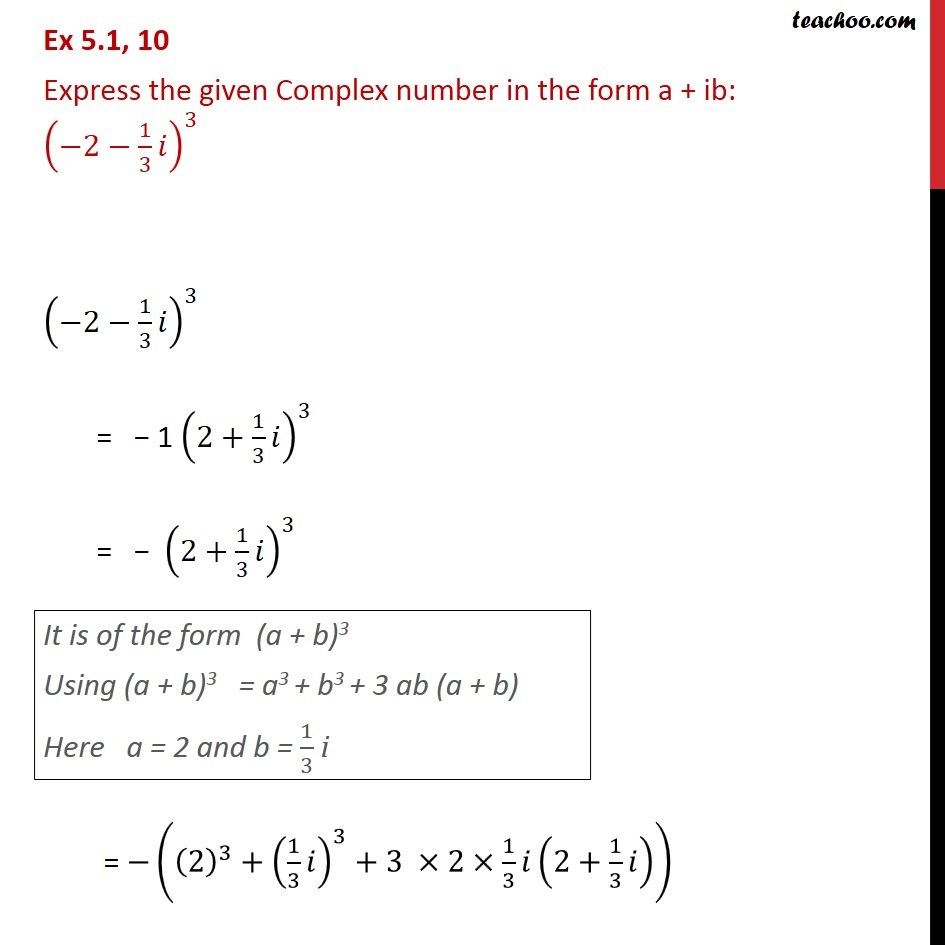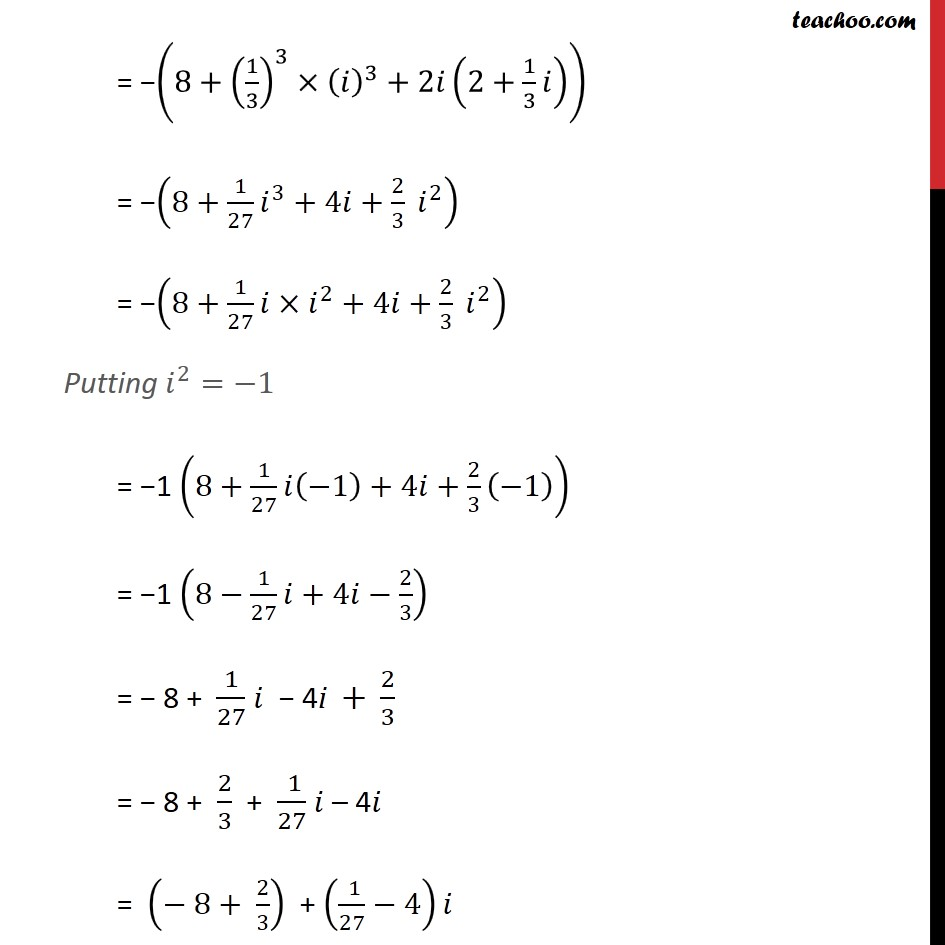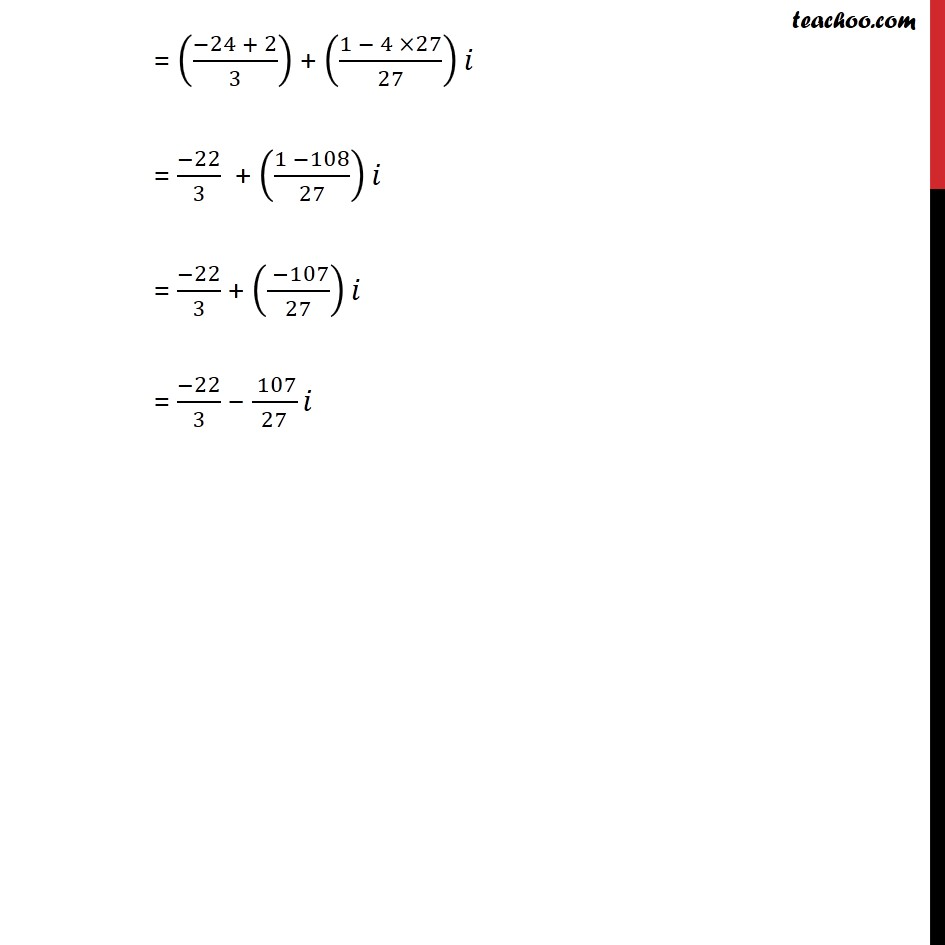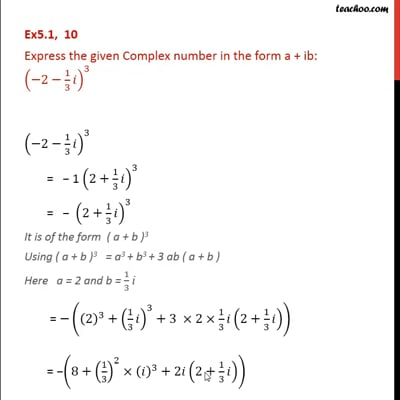Chapter 5 Class 11 Complex Numbers

Class 11
Important Questions for exams Class 11This video is only available for Teachoo black users

Solve all your doubts with Teachoo Black (new monthly pack available now!)

### Transcript

Ex 5.1, 10 Express the given Complex number in the form a + ib: (−2−1/3 𝑖)^3 (−2−1/3 𝑖)^3 = − 1 (2+1/3 𝑖)^3 = − (2+1/3 𝑖)^3 It is of the form (a + b)3 Using (a + b)3 = a3 + b3 + 3 ab (a + b) Here a = 2 and b = 1/3 i = −((2)^3+(1/3 𝑖)^3+3 ×2×1/3 𝑖(2+1/3 𝑖)) = −(8+(1/3)^3×(𝑖)^3+2𝑖(2+1/3 𝑖)) = −(8+1/27 𝑖^3+4𝑖+2/3 𝑖^2 ) = −(8+1/27 〖𝑖×𝑖〗^2+4𝑖+2/3 𝑖^2 ) Putting 𝑖^2=−1 = −1 (8+1/27 𝑖(−1)+4𝑖+2/3 (−1)) = −1 (8−1/27 𝑖+4𝑖−2/3) = − 8 + 1/27 𝑖 − 4i + 2/3 = − 8 + 2/3 + ( 1)/27 𝑖 – 4𝑖 = (− 8+ 2/3) + (( 1)/27−4)𝑖 = ((−24 + 2)/3) + ((1 − 4 ×27)/27)𝑖 = (−22)/3 + ((1 −108)/27)𝑖 = (−22)/3 + (( −107)/27)𝑖 = (−22)/3 − ( 107)/27 𝑖